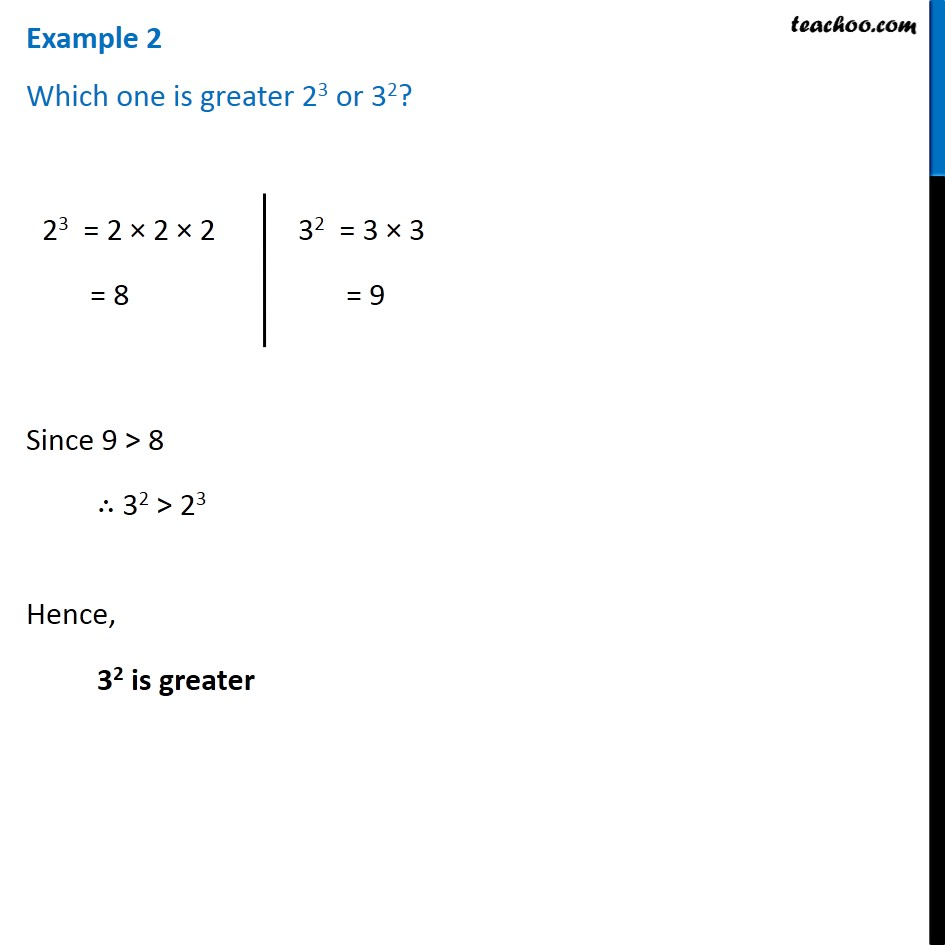1. Chapter 13 Class 7 Exponents and Powers
2. Serial order wise
3. Examples

Transcript

Example 2 Which one is greater 23 or 32? 23 = 2 × 2 × 2 = 8 32 = 3 × 3 = 9 Since 9 > 8 ∴ 32 > 23 Hence, 32 is greater

Examples